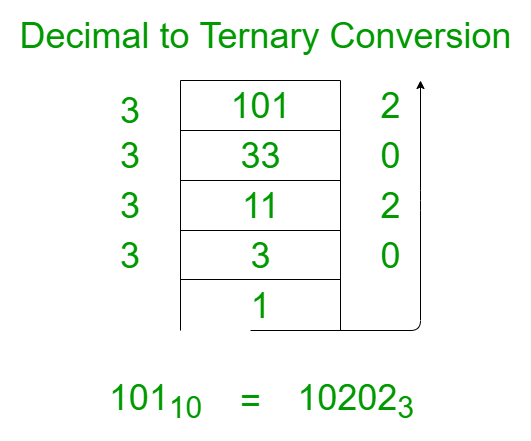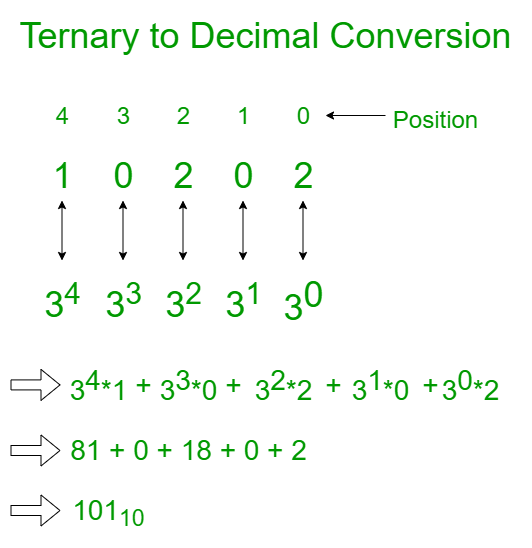# Ternary number system or Base 3 numbers

• Difficulty Level : Medium
• Last Updated : 18 Nov, 2021

A number system can be considered as a mathematical notation of numbers using a set of digits or symbols. In simpler words, the number system is a method of representing numbers. Every number system is identified with the help of its base or radix.

For example, Binary, Octal, Decimal and Hexadecimal Number systems are used in microprocessor programming. In this article, one such number system is discussed.

Ternary Number System:
If the Base value of a number system is 3, then this representation is known as a ternary representation. The digits in this system are 0, 1, and 2
There is also a number system called Balanced Ternary which comprises the digits −1, 0 and +1. The Ternary representation of a number is compact than of binary number system.

Steps to Convert Decimal to Ternary:

1. Divide the number by 3.
2. Get the integer quotient for the next iteration.
3. Get the remainder for the ternary digit.
4. Repeat the steps until the quotient is equal to 0.

For example: let N = 101. The following image illustrates the step by step conversion of 10110 to the base-3.Below is the implementation for the Decimal to Binary and Vice-Versa:

## C++

 `// C++ program to convert decimal``// number to ternary number` `#include ``#include ``#include ``using` `namespace` `std;` `// Function to convert a decimal``// number to a ternary number``void` `convertToTernary(``int` `N)``{``    ``// Base case``    ``if` `(N == 0)``        ``return``;` `    ``// Finding the remainder``    ``// when N is divided by 3``    ``int` `x = N % 3;``    ``N /= 3;``    ``if` `(x < 0)``        ``N += 1;` `    ``// Recursive function to``    ``// call the function for``    ``// the integer division``    ``// of the value N/3``    ``convertToTernary(N);` `    ``// Handling the negative cases``    ``if` `(x < 0)``        ``cout << x + (3 * -1);``    ``else``        ``cout << x;``}` `// Function to convert the decimal to ternary``void` `convert(``int` `Decimal)``{``    ``cout << ``"Ternary number of "``         ``<< Decimal << ``" is: "``;` `    ``// If the number is greater``    ``// than 0, compute the``    ``// ternary representation``    ``// of the number``    ``if` `(Decimal != 0) {``        ``convertToTernary(Decimal);``    ``}``    ``else``        ``cout << ``"0"` `<< endl;``}` `// Driver code``int` `main()``{``    ``int` `Decimal = 2747;` `    ``convert(Decimal);` `    ``return` `0;``}`

## Java

 `// Java program to convert decimal``// number to ternary number``import` `java.io.*;`` ` `class` `GFG``{` `// Function to convert a decimal``// number to a ternary number``static` `void` `convertToTernary(``int` `N)``{``    ``// Base case``    ``if` `(N == ``0``)``        ``return``;` `    ``// Finding the remainder``    ``// when N is divided by 3``    ``int` `x = N % ``3``;``    ``N /= ``3``;``    ``if` `(x < ``0``)``        ``N += ``1``;` `    ``// Recursive function to``    ``// call the function for``    ``// the integer division``    ``// of the value N/3``    ``convertToTernary(N);` `    ``// Handling the negative cases``    ``if` `(x < ``0``)``        ``System.out.print( x + (``3` `* -``1``));``    ``else``        ``System.out.print( x);``}` `// Function to convert the decimal to ternary``static` `void` `convert(``int` `Decimal)``{``    ``System.out.print(``"Ternary number of "`  `+Decimal +``" is: "``);` `    ``// If the number is greater``    ``// than 0, compute the``    ``// ternary representation``    ``// of the number``    ``if` `(Decimal != ``0``) {``        ``convertToTernary(Decimal);``    ``}``    ``else``        ``System.out.println(``"0"` `);``}` `// Driver Code``public` `static` `void` `main (String[] args)``{``    ``int` `Decimal = ``2747``;` `    ``convert(Decimal);` `}``}` `// This code is contributed by shivanisinghss2110`

## Python3

 `# Python3 program to convert decimal``# number to ternary number` `# Function to convert a decimal``# number to a ternary number``def` `convertToTernary(N):``    ` `    ``# Base case``    ``if` `(N ``=``=` `0``):``        ``return``;` `    ``# Finding the remainder``    ``# when N is divided by 3``    ``x ``=` `N ``%` `3``;``    ``N ``/``/``=` `3``;``    ``if` `(x < ``0``):``        ``N ``+``=` `1``;` `    ``# Recursive function to``    ``# call the function for``    ``# the integer division``    ``# of the value N/3``    ``convertToTernary(N);` `    ``# Handling the negative cases``    ``if` `(x < ``0``):``        ``print``(x ``+` `(``3` `*` `-``1``), end ``=` `"");``    ``else``:``        ``print``(x, end ``=` `"");`  `# Function to convert the``# decimal to ternary``def` `convert(Decimal):``    ` `    ``print``(``"Ternary number of "``, Decimal,``          ``" is: "``, end ``=` `"");` `    ``# If the number is greater``    ``# than 0, compute the``    ``# ternary representation``    ``# of the number``    ``if` `(Decimal !``=` `0``):``        ``convertToTernary(Decimal);``    ``else``:``        ``print``(``"0"``, end ``=` `"");` `# Driver Code``if` `__name__ ``=``=` `'__main__'``:``    ` `    ``Decimal ``=` `2747``;` `    ``convert(Decimal);` `# This code is contributed by Rajput-Ji`

## C#

 `// C# program to convert decimal``// number to ternary number``using` `System;` `class` `GFG``{` `    ``// Function to convert a decimal``    ``// number to a ternary number``    ``static` `void` `convertToTernary(``int` `N)``    ``{``        ``// Base case``        ``if` `(N == 0)``            ``return``;``    ` `        ``// Finding the remainder``        ``// when N is divided by 3``        ``int` `x = N % 3;``        ``N /= 3;``        ``if` `(x < 0)``            ``N += 1;``    ` `        ``// Recursive function to``        ``// call the function for``        ``// the integer division``        ``// of the value N/3``        ``convertToTernary(N);``    ` `        ``// Handling the negative cases``        ``if` `(x < 0)``            ``Console.Write( x + (3 * -1));``        ``else``            ``Console.Write( x);``    ``}` `    ``// Function to convert the decimal to ternary``    ``static` `void` `convert(``int` `Decimal)``    ``{``        ``Console.Write(``"Ternary number of "` `+Decimal +``" is: "``);``    ` `        ``// If the number is greater``        ``// than 0, compute the``        ``// ternary representation``        ``// of the number``        ``if` `(Decimal != 0) {``            ``convertToTernary(Decimal);``        ``}``        ``else``            ``Console.WriteLine(``"0"` `);``    ``}` `    ``// Driver Code``    ``public` `static` `void` `Main (``string``[] args)``    ``{``        ``int` `Decimal = 2747;``    ` `        ``convert(Decimal);``    ` `    ``}``}` `// This code is contributed by AnkitRai01`

## Javascript

 ``

Output:

`Ternary number of 2747 is: 10202202`

Time Complexity: O(log3N)

Auxiliary Space: O(log3N)

Steps to Convert Ternary to Decimal:

1. Connect each digit from the ternary number with its corresponding power of three.
2. Multiply each digit with its corresponding power of three and Add up all of the numbers you got.

For example: let N = 10202. The following image illustrates the step by step conversion of 102023 to the base-10.Below is the implementation for the Decimal to Binary and Vice-Versa:

## C++

 `// C++ program to convert a``// ternary number to decimal number` `#include ``#include ``#include ``using` `namespace` `std;` `// Function to convert a ternary``// number to a decimal number``void` `convertToDecimal(``int` `N)``{``    ``cout << ``"Decimal number of "``         ``<< N << ``" is: "``;` `    ``// If the number is greater than 0,``    ``// compute the decimal``    ``// representation of the number``    ``if` `(N != 0) {` `        ``int` `decimalNumber = 0,``            ``i = 0, remainder;` `        ``// Loop to iterate through``        ``// the number``        ``while` `(N != 0) {``            ``remainder = N % 10;``            ``N /= 10;` `            ``// Computing the decimal digit``            ``decimalNumber += remainder``                             ``* ``pow``(3, i);``            ``++i;``        ``}``        ``cout << decimalNumber << endl;``    ``}``    ``else``        ``cout << ``"0"` `<< endl;``}` `// Driver code``int` `main()``{``    ``int` `Ternary = 10202202;` `    ``convertToDecimal(Ternary);` `    ``return` `0;``}`

## Java

 `// Java program to convert a``// ternary number to decimal number``class` `GFG{` `// Function to convert a ternary``// number to a decimal number``static` `void` `convertToDecimal(``int` `N)``{``    ``System.out.print(``"Decimal number of "` `+``                      ``N + ``" is: "``);` `    ``// If the number is greater than 0,``    ``// compute the decimal``    ``// representation of the number``    ``if` `(N != ``0``)``    ``{``        ``int` `decimalNumber = ``0``,``            ``i = ``0``, remainder;` `        ``// Loop to iterate through``        ``// the number``        ``while` `(N != ``0``)``        ``{``            ``remainder = N % ``10``;``            ``N /= ``10``;` `            ``// Computing the decimal digit``            ``decimalNumber += remainder *``                             ``Math.pow(``3``, i);``            ``++i;``        ``}``        ``System.out.print(decimalNumber + ``"\n"``);``    ``}``    ``else``        ``System.out.print(``"0"` `+ ``"\n"``);``}` `// Driver code``public` `static` `void` `main(String[] args)``{``    ``int` `Ternary = ``10202202``;``    ``convertToDecimal(Ternary);``}``}` `// This code is contributed by Rajput-Ji`

## Python3

 `# Python3 program to convert a``# ternary number to decimal number``import` `math;` `# Function to convert a ternary``# number to a decimal number``def` `convertToDecimal(N):` `    ``print``(``"Decimal number of"``, N, ``"is:"``, end ``=` `" "``);` `    ``# If the number is greater than 0,``    ``# compute the decimal``    ``# representation of the number``    ``if` `(N !``=` `0``):` `        ``decimalNumber ``=` `0``;``        ``i ``=` `0``;``        ``remainder ``=` `0``;` `        ``# Loop to iterate through``        ``# the number``        ``while` `(N !``=` `0``):``            ``remainder ``=` `N ``%` `10``;``            ``N ``=` `N ``/``/` `10``;` `            ``# Computing the decimal digit``            ``decimalNumber ``+``=` `remainder ``*` `math.``pow``(``3``, i);``            ``i ``+``=` `1``;``        ` `        ``print``(decimalNumber);``    ` `    ``else``:``        ``print``(``"0"``);` `# Driver code``Ternary ``=` `10202202``;``convertToDecimal(Ternary);` `# This code is contributed by Code_Mech`

## C#

 `// C# program to convert a ternary``// number to decimal number``using` `System;` `class` `GFG{` `// Function to convert a ternary``// number to a decimal number``static` `void` `convertToDecimal(``int` `N)``{``    ``Console.Write(``"Decimal number of "` `+``                           ``N + ``" is: "``);` `    ``// If the number is greater than ``    ``// 0, compute the decimal``    ``// representation of the number``    ``if` `(N != 0)``    ``{``        ``int` `decimalNumber = 0;``        ``int` `i = 0, remainder;` `        ``// Loop to iterate through``        ``// the number``        ``while` `(N != 0)``        ``{``            ``remainder = N % 10;``            ``N /= 10;` `            ``// Computing the decimal digit``            ``decimalNumber += remainder *``                             ``(``int``)Math.Pow(3, i);``            ``++i;``        ``}``        ``Console.Write(decimalNumber + ``"\n"``);``    ``}``    ``else``        ``Console.Write(``"0"` `+ ``"\n"``);``}` `// Driver code``public` `static` `void` `Main()``{``    ``int` `Ternary = 10202202;``    ` `    ``convertToDecimal(Ternary);``}``}` `// This code is contributed by shivanisinghss2110`

## Javascript

 ``

Output:

`Decimal number of 10202202 is: 2747`

Time Complexity: O(log10N)

Auxiliary Space: O(1)

My Personal Notes arrow_drop_up